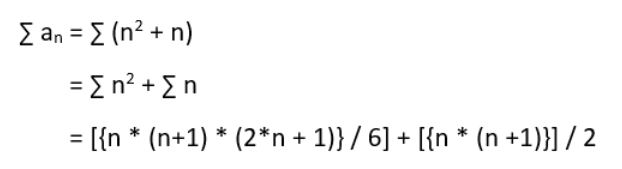# Sum of the series 2 + (2+4) + (2+4+6) + (2+4+6+8) + …… + (2+4+6+8+….+2n)

• Difficulty Level : Easy
• Last Updated : 11 Aug, 2022

Given a positive integer n. The problem is to find the sum of the given series 2 + (2+4) + (2+4+6) + (2+4+6+8) + …… + (2+4+6+8+….+2n), where i-th term in the series is the sum of first i even natural numbers.
Examples:

```Input : n = 2
Output : 8
(2) + (2+4) = 8

Input : n = 5
Output : 70
(2) + (2+4) + (2+4+6) + (2+4+6+8) + (2+4+6+8+10) = 70```

Naive Approach: Using two loops get the sum of each i-th term and then add those sum to the final sum.

## C++

 `// C++ implementation to find the sum``// of the given series``#include `` ` `using` `namespace` `std;`` ` `// function to find the sum``// of the given series``int` `sumOfTheSeries(``int` `n)``{``    ``int` `sum = 0;``    ``for` `(``int` `i = 1; i <= n; i++) {`` ` `        ``// first term of each i-th term``        ``int` `k = 2;``        ``for` `(``int` `j = 1; j <= i; j++) {``            ``sum += k;`` ` `            ``// next term``            ``k += 2;``        ``}``    ``}`` ` `    ``// required sum``    ``return` `sum;``}`` ` `// Driver program to test above``int` `main()``{``    ``int` `n = 5;``    ``cout << ``"Sum = "``         ``<< sumOfTheSeries(n);``    ``return` `0;``}`

## Java

 `// Java implementation to find the``// sum of the given series``class` `GFG{` `    ``// function to find the sum``    ``// of the given series``    ``static` `int` `sumOfTheSeries(``int` `n)``    ``{``        ``int` `sum = ``0``;``        ``for` `(``int` `i = ``1``; i <= n; i++) {``    ` `            ``// first term of each i-th term``            ``int` `k = ``2``;``            ``for` `(``int` `j = ``1``; j <= i; j++) {``                ``sum += k;``    ` `                ``// next term``                ``k += ``2``;``            ``}``        ``}``    ` `        ``// required sum``        ``return` `sum;``    ``}``    ` `    ``// Driver program to test above``    ``public` `static` `void` `main(String[] args)``    ``{``        ``int` `n = ``5``;` `        ``System.out.printf(``"Sum = %d"``,``                     ``sumOfTheSeries(n));``    ``}``}` `// This code is contributed by``// Smitha Dinesh Semwal`

## Python3

 `# Python3 implementation to find``# the sum of the given series` `# function to find the sum``# of the given series``def` `sumOfTheSeries(n):` `    ``sum` `=` `0``    ``for` `i ``in` `range``(``0``, n ``+` `1``):``        ``# first term of each i-th``        ``# term``        ``k ``=` `2``        ``for` `j ``in` `range``(``1``, i ``+` `1``):``            ``sum` `=` `sum` `+` `k;` `            ``# next term``            ``k ``=` `k ``+` `2``    ` `    ``# required sum``    ``return` `sum``;` `# Driver program to test above``n ``=` `5``ans ``=` `sumOfTheSeries(n);``print` `(ans)` `# This code is contributed by saloni1297.`

## C#

 `// C# implementation to find the``// sum of the given series``using` `System;` `class` `GFG{` `    ``// function to find the sum``    ``// of the given series``    ``static` `int` `sumOfTheSeries(``int` `n)``    ``{``        ``int` `sum = 0;``        ``for` `(``int` `i = 1; i <= n; i++) {``    ` `            ``// first term of each i-th term``            ``int` `k = 2;``            ``for` `(``int` `j = 1; j <= i; j++) {``                ``sum += k;``    ` `                ``// next term``                ``k += 2;``            ``}``        ``}``    ` `        ``// required sum``        ``return` `sum;``    ``}``    ` `    ``// Driver program to test above``    ``public` `static` `void` `Main()``    ``{``        ``int` `n = 5;` `        ``Console.Write(``"Sum = "``+``                    ``sumOfTheSeries(n));``    ``}``}` `// This code is contributed by``// vt_m`

## PHP

 ``

## Javascript

 ``

Output:

`Sum = 70`

Efficient Approach:
Let an be the n-th term of the given series.

```an = (2 + 4 + 6 + 8 +....+ 2n).
= sum of first n even numbers.
= n * (n + 1).
= n2 + n.```

Refer this post for the proof of above formula.
Now,Refer this and this post for the proof of above formula.

## C++

 `// C++ implementation to find the sum``// of the given series``#include ``using` `namespace` `std;`` ` `// function to find the sum``// of the given series``int` `sumOfTheSeries(``int` `n)``{``    ``// sum of 1st n natural numbers``    ``int` `sum_n = (n * (n + 1) / 2);``    ` `    ``// sum of squares of 1st n natural numbers``    ``int` `sum_sq_n = (n * (n + 1) / 2) *``                      ``(2 * n + 1) / 3;``                  ` `    ``// required sum``    ``return` `(sum_n + sum_sq_n);``}`` ` `// Driver program to test above``int` `main()``{``    ``int` `n = 5;``    ``cout << ``"Sum = "``         ``<< sumOfTheSeries(n);``    ``return` `0;``}`

## Java

 `// Java implementation to find the``// sum of the given series``class` `GFG{``    ` `    ``// function to find the sum``    ``// of the given series``    ``static` `int` `sumOfTheSeries(``int` `n)``    ``{``        ` `        ``// sum of 1st n natural numbers``        ``int` `sum_n = (n * (n + ``1``) / ``2``);``        ` `        ``// sum of squares of 1st n natural``        ``// numbers``        ``int` `sum_sq_n = (n * (n + ``1``) / ``2``) *``                        ``(``2` `* n + ``1``) / ``3``;``                    ` `        ``// required sum``        ``return` `(sum_n + sum_sq_n);``    ``}``    ` `    ``// Driver program to test above``    ``public` `static` `void` `main(String[] args)``    ``{``        ``int` `n = ``5``;``        ` `        ``System.out.printf(``"Sum = %d"``,``                    ``sumOfTheSeries(n));``    ``}``}` `// This code is contributed by``//Smitha Dinesh Semwal`

## Python3

 `# Python3 implementation to find``# the sum of the given series` `# function to find the sum``# of the given series``def` `sumOfTheSeries(n):` `    ``# sum of 1st n natural numbers``    ``sum_n ``=` `int``((n ``*` `(n ``+` `1``) ``/` `2``));``    ` `    ``# sum of squares of 1st n natural numbers``    ``sum_sq_n ``=` `int` `((n ``*` `(n ``+` `1``) ``/` `2``) ``*` `(``2` `*` `n ``+` `1``) ``/` `3``)``                    ` `    ``# required sum``    ``return` `(sum_n ``+` `sum_sq_n);` `# Driver program to test above``n ``=` `5``ans ``=` `sumOfTheSeries(n)``print` `(ans)` `# This code is contributed by saloni1297.`

## C#

 `// C# implementation to find the``// sum of the given series``using` `System;` `class` `GFG{``    ` `    ``// function to find the sum``    ``// of the given series``    ``static` `int` `sumOfTheSeries(``int` `n)``    ``{``        ` `        ``// sum of 1st n natural numbers``        ``int` `sum_n = (n * (n + 1) / 2);``        ` `        ``// sum of squares of 1st n``        ``// natural numbers``        ``int` `sum_sq_n = (n * (n + 1) / 2) *``                        ``(2 * n + 1) / 3;``                    ` `        ``// required sum``        ``return` `(sum_n + sum_sq_n);``    ``}``    ` `    ``// Driver program to test above``    ``public` `static` `void` `Main()``    ``{``        ``int` `n = 5;``        ` `        ``Console.Write(``"Sum = "``+``                    ``sumOfTheSeries(n));``    ``}``}` `// This code is contributed by``// vt_m`

## PHP

 ``

## Javascript

 ``

Output:

`Sum = 70`

Time complexity: O(1) as it is performing constant operations

Auxiliary space: O(1)

My Personal Notes arrow_drop_up